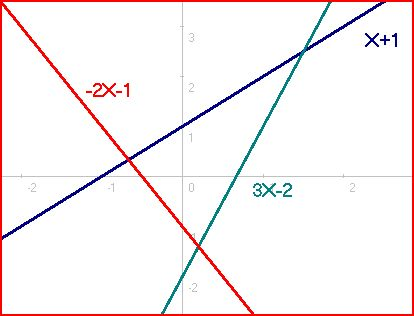# Is something sketchy going on here?

Algebra Level 4The system of equations $4a^4+36a^2b^2+9b^4=20a^2+30b^2-1$ $2a^3b+3ab^3=5ab$ has $n$ nonnegative (meaning both $a$ and $b$ are nonnegative) real solutions. Let these solutions be $(a_1,b_1),(a_2,b_2),\cdots (a_n,b_n)$. The value of the sum $\sum_{i=1}^n a_i+b_i$ can be expressed in the form $\dfrac{a+b\sqrt{c}}{d}$ where $\gcd (a,b,d)=1$ and $c$ is squarefree. Find $a+b+c+d$.

×